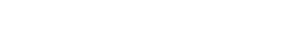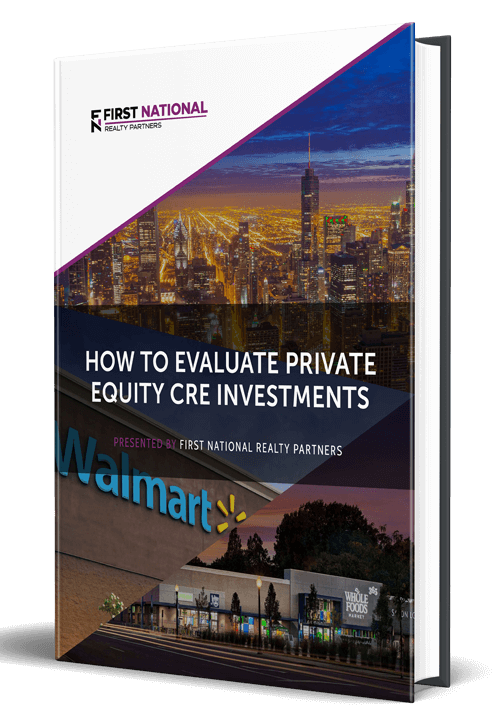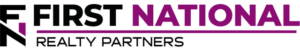# Understanding the Cost Approach to Commercial Real Estate Valuation

### Key Takeaways

• The Cost Approach is one of three commonly used real estate valuation methods.  With it, an appraiser or analyst attempts to estimate the value of a real estate property based on the cost it would take to build it from scratch.
• With the cost approach, the formula used to calculate value is land value plus construction costs, minus accumulated depreciation.  There are specific techniques that are used to calculate construction costs and accumulated depreciation.  They range from basic estimates to detailed line item evaluations.
• As a valuation methodology, the cost approach is most useful when valuing a new real estate property, one that has some special use, or those that are involved in an insurance claim.

At First National Realty Partners, we have a saying that “you make your money on the buy.”  This means that the price paid for a real estate property is one of the largest factors in the return that it ultimately produces.  So, we are very methodical in our approach to calculating a property’s value and very disciplined about the prices that we offer.

In commercial real estate, there are three commonly used approaches to determining a property’s value:  the income capitalization approach (income approach), the sales comparison approach, and the cost approach.  When attempting to value a commercial property, it often makes sense to use all three to triangulate around a certain value.

In this article, the methodology behind the Cost Approach is discussed in detail.  Upon completion, readers will have a better understanding of the logic and calculations behind the cost approach.

In our own real estate investments, we always use the cost approach as part of our pre-purchase due diligence to ensure that we maximize our opportunity to “make money on the buy.”  To learn more about our current commercial real estate investment offerings, click here

## What is the Cost Approach?

The Cost Approach is a methodology that seeks to determine a real estate property’s market value by estimating the cost that it would take to replace it.  The logic behind this states that a reasonable buyer wouldn’t pay more for a property that it would cost to build it from scratch.

## Calculating Value Using the Cost Approach Formula

The cost approach formula used to calculate the value of a property is very straightforward:

Current Market Value = Land Value + (Construction Cost – Accumulated Depreciation)

Let’s review each one of these variables in detail.

### Land Value

A property’s land value is fairly self-explanatory.  It represents the cost that would be required to purchase a parcel of land with a similar size and location.

### Construction Costs

The construction cost is also fairly self-explanatory.  It represents the cost that it would take to build a similar property with similar function.  But the method used to estimate construction costs is much more nuanced.

Typically, there are two approaches used to estimate construction costs.  The Replacement Cost approach estimates the current cost that would be required to construct a building with the same utility, with the same construction materials, and utilizing current construction materials, designs, standards, and layouts.  The Reproduction Cost approach estimates the cost that it would take to construct an exact replica of the property with the original materials, designs, methods, and standards used when it was constructed.  When a property is new, the replacement cost and reproduction cost are nearly identical.  But, as a property ages and new advances are made in construction practices, this gap will grow.

To break down these approaches even further, there are up to four different methods that could be used to calculate replacement or reproduction costs:

1. Comparative Unit Method:  With this method, costs are calculated based on a general per square foot cost.  So, if a real estate property was 10,000 SF and the per SF cost estimate was \$200, construction costs would be estimated at \$2,000,000.
2. Segregated Cost Method:  Rather than lump all cost categories into one bucket, the segregated cost method groups expenses into major categories and estimates the cost for each.  For example, the costs could be grouped into categories like: flooring, electrical, plumbing, and HVAC.  Each of the major groups would have their own cost and the sum of all groups would equal the total cost for the building.
3. Unit In Place Method:  The “unit in place” method provides another layer of detail in the cost estimate process.  With this method, costs are first grouped into major categories and then sub-grouped into smaller categories.  For example, plumbing could be a major category, but the sub-categories could be things like pipes, toilets, faucets, sinks, etc.  The sum of all categories equals the total construction cost.
4. Quantity Survey Method:  This is the most accurate and detailed method for calculating construction costs.  It uses line item detail for every item needed to reproduce or replace the real estate property.  The sum of all line items equals the total cost.

Estimating the construction cost is the most time consuming part of using the cost approach to valuation.

### Accumulated Depreciation

Depreciation is an accounting concept that allows a property owner to “expense” a portion of the property’s value each year to account for its physical deterioration.  This expense shows up as a line item on the property’s income statement and is subtracted as part of the Net Operating Income (NOI) calculation.  Over time, depreciation builds up and results in a difference between the cost of new construction and a property in its current state.  There are three methods that appraisers/analysts can use to estimated the amount of depreciation that a property has accumulated over time:

1. The Age-Life Method:  This is the easiest and most straightforward method used to estimate depreciation.  With it, total age, effective age, and remaining useful life are estimated.  A cost is then assigned to depreciation.
2. The Breakdown Method:  Although this is the most accurate method of measuring accumulated depreciation, it is also the most time consuming.  With it, the appraiser/analyst must measure all forms of depreciation (external, functional, and physical) and add them together to arrive at total accrued depreciation
3. The Market Extraction Method:  This method uses comparable sales to estimate the appropriate depreciation percentage to apply to a subject property.

Once the total amount of accumulated depreciation is estimated, it can be plugged into the valuation equation.

### Valuation Example

To illustrate how all of the above variables come together to estimate the value of a property, an example is helpful.  Suppose that an appraiser has been hired to assess the value of a 35,000 SF retail shopping center.  In their report, they use all three of the major valuation methods, of which the cost approach is one.  The property is 10 years old, so right away, they know that the value of the property must account for some amount of accumulated depreciation.

The value of the land upon which the property sits is estimated to be \$1,000,000, the total construction costs required to construct an equivalent building are estimated to be \$3,000,000, and the property has an estimated total accumulated depreciation of \$750,000.  As such, the property valuation using the cost approach is:

Value = \$1,000,000 + (\$3,000,000 – \$750,000) = \$3,250,000.

## When is the Cost Approach Useful?

Depending on the property type, market conditions, or property age, there may be one valuation methodology that is more useful than another.  With regard to the cost approach, there are three situations in which it is particularly helpful:  new construction, special use properties, and adjusting insurance claims.

### New Construction

When a property is new or like new, the cost approach is helpful because the cost of the materials is recent and there is little to no accumulated depreciation to account for.

### Special Use Properties

A special use property tends to have some unique use or feature that makes it difficult for an appraiser to find comparable properties and/or recent sales.  In such cases, the cost approach to valuation is helpful under the previously mentioned logic that a rational buyer would not pay more for a property than it would take to construct it new.

### Insurance Claims

In a typical insurance policy, only the value of a property’s improvements is insurable.  Because the cost approach separates the value of improvements from land, it is helpful when underwriting insurance policies or adjusting damage claims.

## Pros and Cons Of Using the Cost Approach

The major advantage of the cost approach is that it is relatively simple and prices for construction materials are readily available.

However, it requires a number of estimates, particularly for land, which may make it less accurate than the sales comparison method or income capitalization approach.

## Summary & Conclusion

In real estate investing, the Cost Approach is one of three commonly used real estate valuation methods.  With it, an appraiser or analyst attempts to estimate the value of a property based on the cost it would take to build it from scratch.

With the cost approach, the formula used to calculate value is land value plus construction costs, minus accumulated depreciation.  There are specific techniques that are used to calculate construction costs and accumulated depreciation.  They range from basic estimates to detailed line item evaluations.

As a valuation methodology, the cost approach is most useful when valuing a new property, one that has some special use, or those that are involved in an insurance claim.

## Interested In Learning More?

First National Realty Partners is one of the country’s leading private equity commercial real estate investment firms. With an intentional focus on finding world-class, multi-tenanted assets well below intrinsic value, we seek to create superior long-term, risk-adjusted returns for our investors while creating strong economic assets for the communities we invest in.

Get instant access to all of our current and past commercial real estate deals.## Subscribe Now

Get the latest news on real estate

FNRP Spotlight Series

1031 Exchange

Acquisitions

## Get More From FNRP## How to Evaluate Private Equity CRE Investments## How to Complete a 1031 Exchange with a Private Equity Sponsor